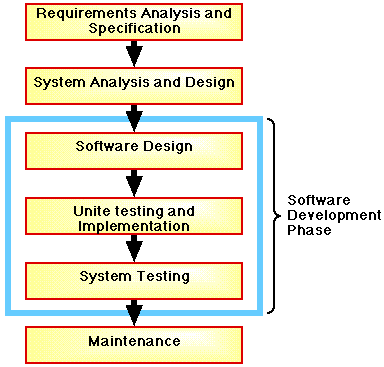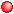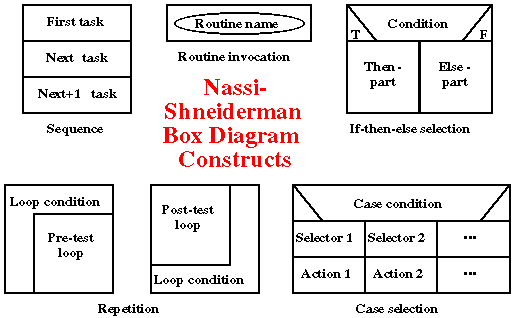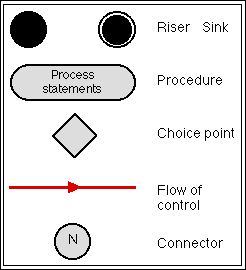# NOTE: This set of www pages is not the set of www pages for the curent version of COMP101. The pages are from a previous version that, at the request of students, I have kept on line.

## CONTENTS

 1. Design 2. Nassi-Shneiderman charts 3. Activity Diagrams (ADs) 4. Design guidance rules 5. Style review 5.1. Indentation 5.2. Comments ` ` 5.3. Naming conventions 5.4. Constants 5.5. Declaration order 6. Efficiency 6.1. Code efficiency 6.2. Memory efficiency 7. Some standard methods

## 1. DESIGN

The design, implementation and unit testing and system testing stages of the overall software life cycle collectively describes the software development stage (the area enclosed in a box in Figure1).Figure 1: Stages in the "classic" lifecycle of software

The first phase in software development is design. Design may be described as:

".. the process of applying various techniques and principles for the purpose of defining a device, a process or a system in sufficient detail to permit its physical realisation"
` `

In our case we use techniques and principles such as:Object oriented analysisClass diagramSummary tablesNassi-Shneiderman chartsActivity diagrams

The thing we wish to design is a computer software system, and its eventual physical realisation will be in the form of a program written in a programming language (e.g. Java).

An alternative view of design is to consider the process to be a modelling process whereby a model of the final realisation is produced.

Whatever the case the design process combines:

1. Intuition and judgement based on previous experience.
2. A set of principles and/or "rules of thumb" (heuristics) to act a guidance.
3. A set of criteria whereby quality can be judged - requirements, readability/understandability.
4. A process of iteration.

## 2. NASSI-SHNEIDERMAN CHARTS

 Many design techniques/tools are based on graphical representations ("a picture tells a thousand words"). More precisely many design tools use box diagrams. As the name suggests box diagrams use shapes such as rectangles or squares to represent components in a design. One such tool is the Nassi-Shneiderman chart (some people call them diagrams). The charts were first proposed by Ike Nassi and Ben Shneiderman in the early 1970s (Nassi and Shneiderman 1973) and later extended by others (Woodward 1987). A reminder of the graphical representation of the control constructs using N-S charts is given in Figure 2. Note the following: To represent sequence, two boxes are connected bottom to top. ` ` To represent an "if-then-else" , a conditional box is followed by a "then part" and an "else part" box. Repetition is depicted with a bounding pattern that encloses the process ("do-while part" or "repeat-until part") to be repeated. Selection is shown by a "case-condition" box followed by a set of "selection" boxes each associated with an "action" box. A method (routine) name within an ellipse (within a box) is used to indicate a reference call to the named procedure/function. A number of Nassi-Shneiderman chart editors are available over the WWW. Nassi-Shneiderman links:Figure 2: Nassi-Shneiderman chart constructs

 Activity Diagrams (ADs) are the UML variation of the well established Control Flow Diagrams (CFD) used in the imperative paradigm. The CFD is the most widely used graphical representation for procedural design. The main advantage is that it clearly shows the flow of control (i.e. the "paths") through a software systems. The constructs used to produce flow charts are presented in Figure 3. ` `Figure 3: Control Flow (Action) Diagram constructs

## 4. DESIGN GUIDANCE RULES

 The design should be completely broken down into classes with fields and methods. High level methods should invoke low level methods. Low level methods perform a single task. Be suspicious of incomplete decomposition if a method requires more than 15-20 lines of code to implement. Designs should NOT include duplicate code. Code that is similar to code in another place should be combined so the code is written only once. If necessary use parameters to accommodate differences in similar code segments. ` ` Follow principles of structured programming. One entry - one exit control structures. Enter at the top, exit at the bottom. Wherever possible refrain from using "exit" statements (break) in loops. Use appropriate features of the language where applicable. For example, use switchstatements instead of multiple "ifs". It is usually bad style to assign the result of a Boolean function to a Boolean variable, or compare the result of a Boolean function to a Boolean constant.

## 6. EFFICIENCY

A well engineered piece of software should make efficient use of critical resources, particularly:

1. Processing power
2. Primary memory storage

However, efficiency concerns should not generally override the need for clarity, readability and correctness.

### 6.1 Code efficiency

Code efficiency is generally measured in terms of the number of machine statements that need to be processed. Note that:

1. A program statement in a high level language such as Java may require many machine code statements to implement it.
` `
1. Different high-level statements will usually have different numbers of machine code statements associated with them.
2. However, generally speaking the number of high level language statements making up an algorithm is directly proportional to processing efficiency.
3. The number of statements required to process an algorithm is not necessarily the same as the number of lines of code required to define an algorithm - particularly when using loops.

### 6.2 Memory Efficiency

For most computers memory restrictions are a thing of the past. This is not the case for embedded systems.

## 7. SOME STANDARD METHODS

There are some "standard" methods that are used repeatedly by Java programmers. These are so common that they have been given names, the most common are "set" and "get" methods. A "set" method is a public method which is used to assign a value to a private instance or class field. A "get" method is a public method which is used to return the value of a private instance or class field. An illustration of the use of these methods is given in Tables 1 and 2.

 ```// Common Methods Program // Frans Coenen // 24 March 2000 // Dept Computer Science, University of Liverpool class CommonMethods { // ------------------ FIELDS ------------------------ private int value1=0, value2=0; // ------------------ METHODS ------------------------ /* Get methods */ public int getValue1() { return(value1); } public int getValue2() { return(value2); } /* Set methods */ public void setValue1(int value) { value1 = value; } public void setValue2(int value) { value2 = value; } } ```

Table 1: Use of "set" and "get" methods

 ```// Common Methods Application Program // Frans Coenen // 24 March 2000 // Dept Computer Science, University of Liverpool class CommonMethodsApp { // ------------------ METHODS ------------------------ /* Main method */ public static void main(String[] args) { // Create two instances of CommonMethods Class CommonMethods instance1 = new CommonMethods(); CommonMethods instance2 = new CommonMethods(); // Set values instance1.setValue1(11); instance1.setValue2(12); instance2.setValue1(21); instance2.setValue2(22); // Calculation using get methods System.out.println("Total of value1s: = " + instance1.getValue1() + "+" + instance2.getValue1() + " = " + (instance1.getValue1()+instance2.getValue1())); System.out.println("Total of value2s: = " + instance1.getValue2() + "+" + instance2.getValue2() + " = " + (instance1.getValue2()+instance2.getValue2())); } } ```

Table 2: Application class for code presented in Table 1

 ```Total of value1s: = 11+21 = 32 Total of value2s: = 12+22 = 34 ```

Table 3: Sample output from code presented in Table 2

Another standard method is the toString method. The toString method is defined in the class Object. All other class (both those defined in the Java API and those created by the programmer) are sub-classes of the class Object. Consequently the toString method is inherited (either directly or indirectly) by all other classes. The toString method is typically used in output statements to convert an instance of a class, identified by its name, to a string representation; the resulting representation comprising the class name and the address of the instance in memory. Thus, for example, if we included the line:

 ```// Output using toString method System.out.println(instance1 + "\n" + instance2); ```

at the end of the main method in the application class presented in Table 2 we would get output of the form:

 ```Total of value1s: = 11+21 = 32 Total of value2s: = 12+22 = 34 CommonMethods@360be0 CommonMethods@45a877 ```

Usually the toString method is overridden to produce some more meaningful output. For example we might include the following in our CommonMethods class presented in Table 1:

 ```/* ToString method */ public String toString() { return("Value1 = " + value1 + ", Value2 = " + value2); } ```

In which case the output produced by our revised version of the CommonMethodsApp application class would be:

 ```Total of value1s: = 11+21 = 32 Total of value2s: = 12+22 = 34 Value1 = 11, Value2 = 12 Value1 = 21, Value2 = 22 ```

## REFERENCES

Created and maintained by Frans Coenen. Last updated 10 February 2015